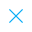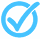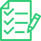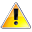Call Support +91-85588-96644

Keep me logged in
You can't leave Captcha Code empty
By submitting this form, you agree to the Terms & Privacy Policy.
ORTests givenPair of Linear Equations in Two Variables - 1

Questions

Time

Highest
score

Level

ENGLISH

Language

Topics Covered:MathematicsSAT (Science, Math, Social Science)Pair of Linear Equations in Two VariablesWord ProblemsGeometric Representation of a Pair of Linear Equations in 2 VariablesNumber of Solutions of a Pair of Linear EquationsFinding Solution of a Given Linear EquationOther Situational ProblemsPair of Linear Equations in Two VariablesQuadratic Equations

Latest TestsAreas Related to Circles - 2 (Class X)

25 Min - 15 Ques

Take TestProbability - 2 (Class X)

25 Min - 15 Ques

Take TestStatistics - 1 (Class X)

25 Min - 15 Ques

Take TestThis is a concept-building practice test and may not have exact structure as you would expect in the actual exam. Please exercise your discretion to attempt it or go to structured Featured Section.

Description:
Pair of Linear Equations in 2 Variables - 1 is a practice test meant class X students
 Type Comment Question
Topper(s) of this Test
•Swayambhuba

Score: 12/15

•Nitya Setia

Score: 12/15

•Suresh V R

Score: 12/15

•Divyam Sultania

Score: 10/15Related Tests